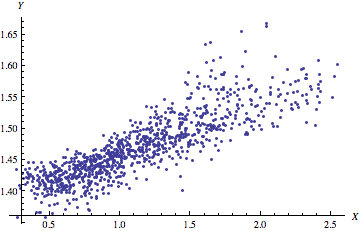Correlation

A correlation is a statistical method used to determine if a relationship exists between variables.  If there is a relationship between the variables itindicates a departure from independence. In other words, the higher the correlation the stronger the relationship and thus the more the variables have in common at least on the surface.

There are four common types of relationships between variables there are the following

1. positive-Both variables increase or decrease in value
2. Negative- One variable decreases in value while another increases.
3. Non-linear-Both variables move together for a time then one decreases while the other continues to increase
4. Zero-No relationship

The most common way to measure the correlation between variables is the Pearson product-moment correlation aka correlation coefficient aka r.  Correlations are usually measured on a standardized scale that ranges from -1 to +1. The value of the number, whether positive or negative, indicates the strength of the relationship.

The Person Product Moment Correlation test confirms if the r is statistically significant or if such a relationship would exist in the population and not just the sample. Below are the assumptions

• Subjects are randomly selected
• Both populations are normally distributed

Here is the process for finding the r.

1. Determine hypotheses
• H0: = 0 (There is no relationship between the variables in the population)
• H0: r ≠ 0 (There is a relationship between the variables in the population)
2. Decided what the level of significance will be
3. Calculate degrees of freedom to determine the t critical value (computer does this)
4. Calculate Pearson’s (computer does this)
5. Calculate t value (computer does this)
6. State conclusion.

Below is an example

A clerk wants to see if there is a correlation between the overall grade students get on an exam and the number of words they wrote for their essay. Below are the results

1                             79                           147
2                             76                           143
3                             78                           147
4                             84                           168
5                             90                           206
6                             83                           155
7                             93                           192
8                             94                           211
9                             97                           209
10                          85                           187
11                          88                           200
12                          82                           150

Step 1: State Hypotheses
H0: There is no relationship between grade and the number of words on the essay
H1: There is a relationship between grade and the number of words on the essay

Step 2: Level of significance
Set to 0.05

Step 3: Determine degrees of freedom and t critical value
t-critical = + 2.228 (This info is found in a chart in the back of most stat books)

Step 4: Compute r
r = 0.93                       (calculated by the computer)

Step 5: Decision rule. Calculate t-value for the r

t-value for r = 8.00  (Computer found this)

Since the computed t-value of 8.00 is greater than the t-critical value of 2.228 we reject the null hypothesis.

Step 6: Conclusion
Since the null hypothesis was rejected, we conclude that there is evidence that a strong relationship between the overall grade on the exam and the number of words written for the essay. To make this practical, the teacher could tell the students to write longer essays if they want a better score on the test.

IMPORTANT NOTE

When a null hypothesis is rejected there are several possible relationships between the variables.

• Direct cause and effect
• The relationship between X and Y may be due to the influence of a third variable not in the model
• This could be a chance relationship. For example, foot size and vocabulary. Older people have bigger feet and also a larger vocabulary. Thus it is a nonsense relationship